Sunday, October 25, 2020
Home > CBSE Class 12 > NCERT Solutions for Class 12 Maths Integration Exercise 7.1

# NCERT Solutions for Class 12 Maths Integration Exercise 7.1# NCERT Solutions for Class 12 Maths Integrations

Hi Students, Welcome to Amans Maths Blogs (AMB). In this post, you will get the NCERT Solutions for Class 12 Maths Integration Exercise 7.1.

NCERT Solutions for class 12 is highly recommended by the experienced teacher for students who are going to appear in CBSE Class 12 and JEE Mains and Advanced and NEET level exams. Here You will get NCERT Solutions for Class 12 Maths Integration Exercise 7.1 of all questions given in NCERT textbooks of class 12 in details with step by step process.

CBSE Class 12th is an important school class in your life as you take some serious decision about your career. And out of all subjects, Maths is an important and core subjects. So CBSE NCERT Solutions for Class 12th Maths is major role in your exam preparation as it has detailed chapter wise solutions for all exercise. This NCERT Solutions can be downloaded in PDF file. The downloading link is given at last.

The PDF books of NCERT Solutions for Class 12 are the first step towards the learning and understanding the each sections of Maths, Physics, Chemistry, Biology as it all help in engineering medical entrance exams. To solve it, you just need to click on download links of NCERT solutions for class 12.

NCERT Solutions for Class 12 Maths are not only the solutions of Maths exercise but it builds your foundation of other important subjects. Getting knowledge of depth concept of CBSE Class 12th Maths like Algebra, Calculus, Trigonometry, Coordinate Geometry help you to understand the concept of Physics and Physical Chemistry.

As we know that all the schools affiliated from CBSE follow the NCERT books for all subjects. You can check the CBSE NCERT Syllabus. Thus, NCERT Solutions helps the students to solve the exercise questions as given in NCERT Books

## NCERT Solutions for Class 12 Maths Integration Exercise 7.1

Find an anti derivative (or integral) of the following functions by the method of inspection.

NCERT Solutions for Class 12 Maths Integration Exercise 7.1: Ques No 1.

sin 2x

NCERT Solutions:NCERT Solutions for Class 12 Maths Integration Exercise 7.1: Ques No 2.

cos 3x

NCERT Solutions: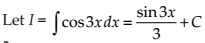NCERT Solutions for Class 12 Maths Integration Exercise 7.1: Ques No 3.

e2x

NCERT Solutions:NCERT Solutions for Class 12 Maths Integration Exercise 7.1: Ques No 4.

(ax + b)2

NCERT Solutions:NCERT Solutions for Class 12 Maths Integration Exercise 7.1: Ques No 5.

sin 2x – 4e3x

NCERT Solutions: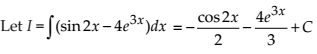Find the following integrals in Exercises 6 to 20:

NCERT Solutions for Class 12 Maths Integration Exercise 7.1: Ques No 6.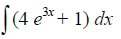NCERT Solutions:NCERT Solutions for Class 12 Maths Integration Exercise 7.1: Ques No 7.NCERT Solutions: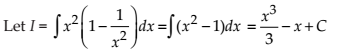NCERT Solutions for Class 12 Maths Integration Exercise 7.1: Ques No 8.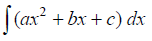NCERT Solutions:NCERT Solutions for Class 12 Maths Integration Exercise 7.1: Ques No 8.NCERT Solutions:NCERT Solutions for Class 12 Maths Integration Exercise 7.1: Ques No 9.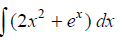NCERT Solutions: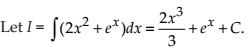NCERT Solutions for Class 12 Maths Integration Exercise 7.1: Ques No 10.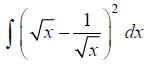NCERT Solutions:NCERT Solutions for Class 12 Maths Integration Exercise 7.1: Ques No 11.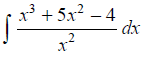NCERT Solutions: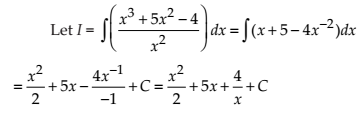NCERT Solutions for Class 12 Maths Integration Exercise 7.1: Ques No 12.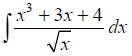NCERT Solutions: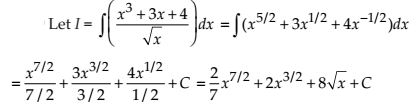NCERT Solutions for Class 12 Maths Integration Exercise 7.1: Ques No 13.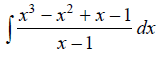NCERT Solutions:NCERT Solutions for Class 12 Maths Integration Exercise 7.1: Ques No 14.NCERT Solutions: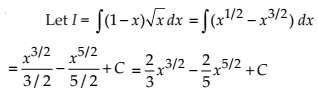NCERT Solutions for Class 12 Maths Integration Exercise 7.1: Ques No 15.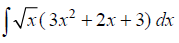NCERT Solutions: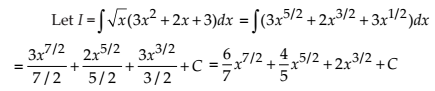NCERT Solutions for Class 12 Maths Integration Exercise 7.1: Ques No 16.NCERT Solutions:NCERT Solutions for Class 12 Maths Integration Exercise 7.1: Ques No 17.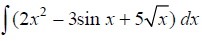NCERT Solutions: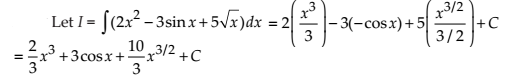NCERT Solutions for Class 12 Maths Integration Exercise 7.1: Ques No 18.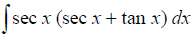NCERT Solutions: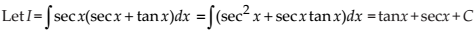NCERT Solutions for Class 12 Maths Integration Exercise 7.1: Ques No 19.NCERT Solutions:NCERT Solutions for Class 12 Maths Integration Exercise 7.1: Ques No 20.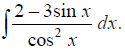NCERT Solutions: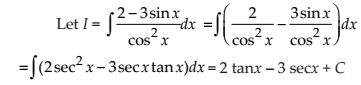NCERT Solutions for Class 12 Maths Integration Exercise 7.1: Ques No 21.

The anti derivative of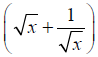equals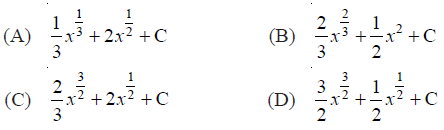NCERT Solutions:NCERT Solutions for Class 12 Maths Integration Exercise 7.1: Ques No 22.

Ifsuch that f (2) = 0. Then f (x) is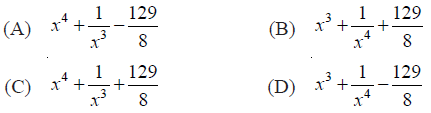NCERT Solutions:

error: Content is protected !!